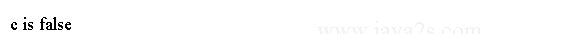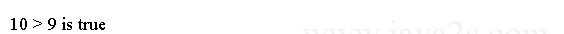# Java Tutorial - Java Relational Operators

Java relational operators determine the relationship between two operands.

## Relational Operators List

The relational operators in Java are:

Operator Result
== Equal to
!= Not equal to
> Greater than
< Less than
>= Greater than or equal to
<= Less than or equal to

For example, the following code fragment is perfectly valid. It compares two int values and assign the result to `boolean` value `c`.

```
public class Main {
public static void main(String[] argv) {
int a = 4;
int b = 1;
boolean c = a < b;

System.out.println("c is " + c);
}
}
```

The result of `a < b` (which is false) is stored in `c`.## Example

The outcome of a relational operator is a boolean value. In the following code, the `System.out.println` outputs the result of a relational operator.

```public class Main {
public static void main(String args[]) {
// outcome of a relational operator is a boolean value
System.out.println("10 > 9 is " + (10 > 9));
}
}
```

The output generated by this program is shown here: